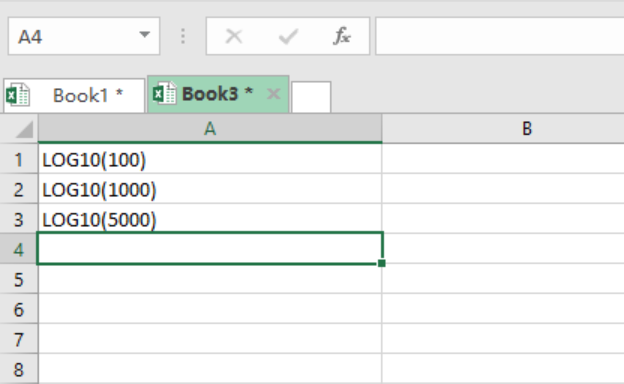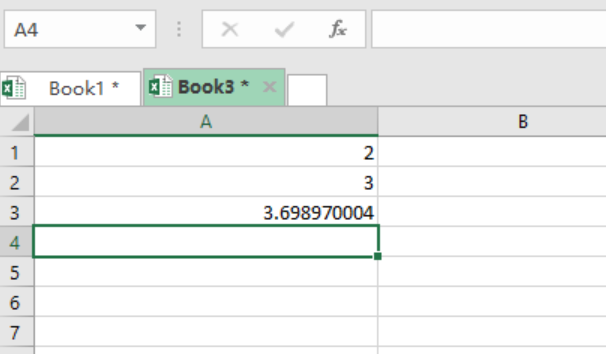Get instant live expert help with Excel or Google Sheets“My Excelchat expert helped me in less than 20 minutes, saving me what would have been 5 hours of work!”

#### Post your problem and you’ll get Expert help in seconds.

Your message must be at least 40 characters
Our professional Expert are available now. Your privacy is guaranteed.

# How to use LOG10 function in Excel

We no longer have to worry about carrying out strenuous mathematical calculations that involve base 10 in excel. With the use of Excel Log10 function, we can be sure to get all the algorithm numbers that the formula is applied to. This article will explain the Log10 function step by step.

## Formula

`=LOG10 (number)`

## Arguments

Number- this specifies the number of which you want its logarithm.

## How the function is used

You need to first understand the parameters or arguments for the formula before you start using it. It is the parameters that will teach you on how to properly use the formula, thus knowing the formula alone isn’t much important.

## Parameters to use the Excel LOG10 function

1.       LOG10 function can be applied to all excel worksheet.
2.       LOG10 function returns a numeric value
3.       The number value in the application should be greater than 0

Note that this function only allows you to get the base 10 of positive numbers.

## ExampleFigure 1: Values for which we want to find logarithm for.

Procedure.

Insert the “Equals to” sign before the LOG10, and then Press Enter.Figure 2: Showing the LOG10 of the specified numbers.

## Notes

There are instances when one will get an error after using the LOG10 function. This can be due to the following two reasons:

#NUM! – This error will occur if you supply a negative or zero number.

#VALUE! – This error will occur if you supply a non-numeric number.

Solution examplesI need a formula that has these 2 rules. If letter was rec'd between 1st and 15th = 1st of the month following letter rec'd date. If letter was rec'd between 16thst and end of the month= 1st of the 2nd month following letter rec'd date.
Solved by V. F. in 20 minsI need a column to total (Hours)(85) + (Travel)(.55) per line
Solved by F. Q. in 20 minsTrying to figure out what is wrong with my formula in Oct-ZD tab in column AY and BG.
Solved by O. Q. in 8 minsSo I have used vlookup to find the price of a product on a different sheet and then I have tried to times that by a quantity. e.g. =vlookup(d4,table35,2,false)*e4 This is the formula I typed in which worked for another list I did but for some reason it's not working now even though everything is practically the same, apart from the contents. Could you help?
Solved by B. U. in 60 minsI have a column containing date and time data together and i need to isolate the time data. When I tried to use text to column, it changed all the times to AM. There is an extremely large amount of data - over 550,000 rows so manually changing it won't work. The file is too large to attach.
Solved by S. E. in 40 mins